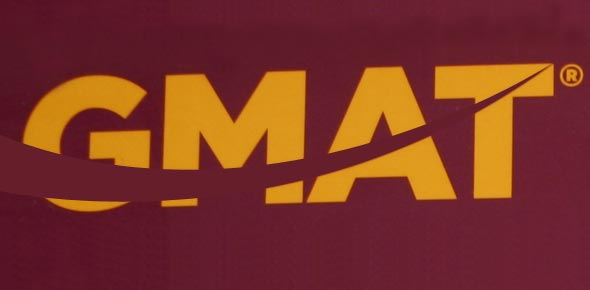# GMAT Math Quiz - 5 Questions In 10 Minutes

10 Questions | Attempts: 350
ShareSettingsThis GMAT quiz will test your understanding of basic concepts in Number Properties, Inequalities within Data Sufficiency, and Rates on the GMAT. It is a timed test. You have 10 minutes to do 5 questions.

• 1.
How many ending zeroes does 30! have?
• A.

2

• B.

3

• C.

5

• D.

7

• E.

9

• 2.
What is the remainder when     is divided by 5?
• A.

0

• B.

1

• C.

2

• D.

3

• E.

4

• 3.
If p is the product of the integers from 1 to 30, inclusive, what is the greatest integer k for which 3k is a factor of p?
• A.

20

• B.

18

• C.

16

• D.

14

• E.

12

• 4.
If two sides of a triangle have lengths of 5 and 8, which of the following could the perimeter of the triangle?             I. 16 II. 20 III. 26
• A.

None

• B.

I only

• C.

II only

• D.

I and II only

• E.

II and III only

• 5.
Chris and James are cycling in the same direction, on the same course. At 3:00 pm., Chris, who is peddling at 20 miles per hour, crosses a bridge. One hour later, James passes the same bridge. James is traveling at 24 miles per hour. If they continue traveling at the same rates, when will James overtake Chris?
• A.

9:30 pm

• B.

9:00 pm

• C.

9:45 pm

• D.

10:00 pm

• E.

8:00 pm

• 6.
What is the remainder when the positive integer n is divided by 3?  1)      The remainder when n is divided by 4 is 1.  2)      The remainder when n + 1 is divided by 6 is 2.
• A.

Statement (1) ALONE is sufficient, but statement (2) alone is not sufficient.

• B.

Statement (2) ALONE is sufficient, but statement (1) alone is not sufficient.

• C.

BOTH statements TOGETHER are sufficient, but NEITHER statement ALONE is sufficient.

• D.

EACH statement ALONE is sufficient.

• E.

Statements (1) and (2) TOGETHER are NOT sufficient.

• 7.
Is
• A.

Statement (1) ALONE is sufficient, but statement (2) alone is not sufficient.

• B.

Statement (2) ALONE is sufficient, but statement (1) alone is not sufficient.

• C.

BOTH statements TOGETHER are sufficient, but NEITHER statement ALONE is sufficient.

• D.

EACH statement ALONE is sufficient.

• E.

Statements (1) and (2) TOGETHER are NOT sufficient.

• 8.
Is  .
• A.

Statement (1) ALONE is sufficient, but statement (2) alone is not sufficient.

• B.

Statement (2) ALONE is sufficient, but statement (1) alone is not sufficient.

• C.

BOTH statements TOGETHER are sufficient, but NEITHER statement ALONE is sufficient.

• D.

EACH statement ALONE is sufficient.

• E.

Statements (1) and (2) TOGETHER are NOT sufficient.

• 9.
In how many different ways can a chairperson and a secretary be selected from a committee of 9 people?
• A.

24

• B.

36

• C.

72

• D.

80

• E.

120

• 10.
If is  an integer?    is an integer. is an integer.
• A.

Statement (1) ALONE is sufficient, but statement (2) alone is not sufficient.

• B.

Statement (2) ALONE is sufficient, but statement (1) alone is not sufficient.

• C.

BOTH statements TOGETHER are sufficient, but NEITHER statement ALONE is sufficient.

• D.

EACH statement ALONE is sufficient.

• E.

Statements (1) and (2) TOGETHER are NOT sufficient.

## Related TopicsBack to top
×

Wait!
Here's an interesting quiz for you.